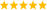# LabVIEW

cancel
Showing results for
Search instead for
Did you mean:

## Re: Sine fit to evenly sampled data

@Bob_Schor

Yes, no problem - I have attached an Excel file with sample data.  At "point A" I will always have at least one period of data, but it will have the same period as that at "point B" and "point C", which is where I need it (so that bit isn't a problem). The problem is programatically finding the period at point A.  In this example, it is clearly 15 minutes, but I will have so much data that I need to fit a sine function to the data programatically.

Message 11 of 17
(763 Views)

## Re: Sine fit to evenly sampled data

A sine function is a periodic function and your data is not. It can be described as a severly truncated sine function.

Do all data set look approximately similar? That would give you pretty strong boundaries on the wavelength (or frequency) or do you sometimes have several periods or even more truncation? Is the amplitude known to be 1 or can it vary? Is there a possible background slope or offset in the data?

In any case, fitting to a sine function given reasonable initial parameter estimates (amplitude, frequency, phase, offset) should work pretty well using nonlinear curve fit. Have you tried?LabVIEW Champion. It all comes together inGCentralWhat does "Engineering Redefined" mean??
Message 12 of 17
(754 Views)

## Re: Sine fit to evenly sampled data

As usual, I completely agree with Altenbach, particularly as he agrees with my suggestion that you fit a sinusoid with offset, amplitude, phase, and frequency (requiring a non-linear fitting algorithm, tricky at times to code but very adept at producing answers quickly).  For such algorithms, starting with good estimates of these parameters is very important.  An excellent starting value for the offset is simply the mean of your data, while a reasonable estimate for amplitude is the max(abs(data - offset)) (where Offset is the constant you just calculated by taking the mean and Data refers to the array of data).  You may already have a good estimate for frequency (in the present example, it was 1/15, 1 divided by the period, ignoring units of time).

Using the data for the A points and using only the first 15 points (and assuming the period is exactly 15 minutes, so I'm analyzing exactly one cycle, which makes the Fourier Series easy to do right on the Excel Worksheet), I get an offset of 0.1, an amplitude of 9.2, and a peak at 4.8 minutes.  Note the key to this Quick Solution was the assumption and comments in parentheses.

Bob Schor

Message 13 of 17
(740 Views)

## Re: Sine fit to evenly sampled data

The non-linear curve fit does seem like the best solution here.  I have attached a 1st draft of the program here (I can add robustness later). Thank you all for your help.

Message 14 of 17
(719 Views)

## Re: Sine fit to evenly sampled data

I got essentially the same answers for this data set using a Simplex method (instead of LevMar) and adding an Offset parameter (your model assumes the offset is 0 -- always better to use a model with fewer parameters, especially if you "know" the value).  Congratulations on pulling this together -- now tackle those data!

Bob Schor

P.S. -- you might consider outputting a final "goodness of fit" number to detect if one of your fits goes "way off" (possibly because you have half a wave of data and it is absurdly noisy, for example).

Message 15 of 17
(712 Views)

## Re: Sine fit to evenly sampled data

@sharon1991 wrote:

The non-linear curve fit does seem like the best solution here.  I have attached a 1st draft of the program here (I can add robustness later). Thank you all for your help.

A few comments:

• index array is resizeabale, so you only need one instance. And if you want the indices in order starting with zero, you don't even need to wire them.
• The x array should depend in the size of the y array, not on a constant.
• Use the VI model for simplicity and speed. Text formulas are inefficient.
• There is a primitive for 1/x
• If you use the formula model, you can use the LM formula string function to calculate the best fit using a finer x-ramp. (Or just use the output from the nonlinear fit).
• ...LabVIEW Champion. It all comes together inGCentralWhat does "Engineering Redefined" mean??
Message 16 of 17
(699 Views)

## Re: Sine fit to evenly sampled data

Try this for some ideas.....LabVIEW Champion. It all comes together inGCentralWhat does "Engineering Redefined" mean??
Message 17 of 17
(686 Views)